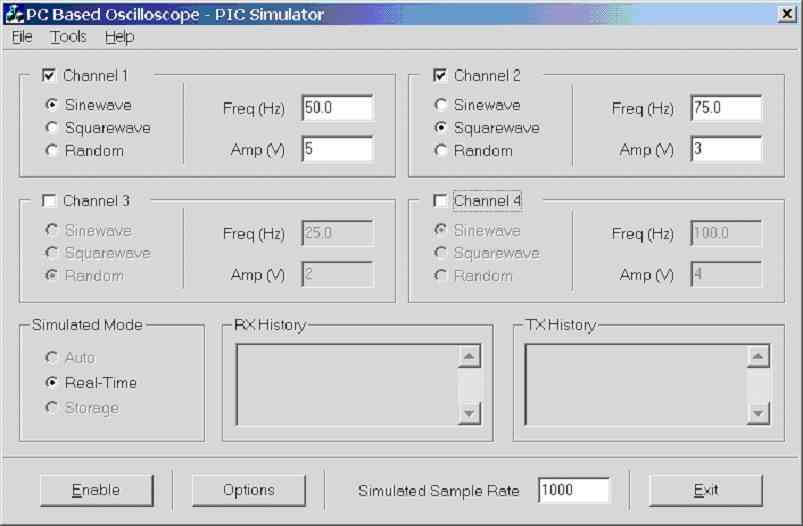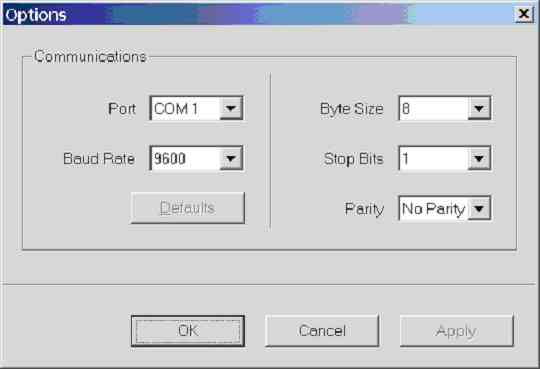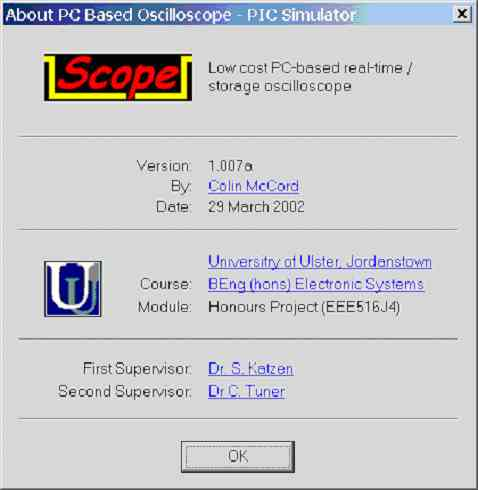8.0. The Simulator Program

This program is a windows dialog based program written in Microsoft Visual C++ 6.0, Figure 8.0a shows a screen dump of the main dialog. The program is easy to use; the user selects the type of waveform, amplitude and frequency for each channel. The PC based scope program will automatically enable / disable channels using the ‘control’ protocol (user can override using the tick boxes beside channel number).Figure 8.0a. Screen dump of sim.exe V1.007a - 29/03/2002

If a channel is disabled all of its controls are greyed (i.e. ch3 and ch4 are disabled in figure 8.0a), notice that the user has the option to specify real-time, storage or auto (controlled by PC). At present ‘auto’ and ‘storage’ mode have not been implemented. There are two large edit boxes that were designed to be used to display RX and TX history in a low level format, for debugging purposes, this feature was not implemented because there were no communication problems during development.

The program was used to test the communication protocols (real-time, storage, control) and graphical scope display (including triggering methods) at different baud rates. Note accurate timing is not simulated as this is difficult to achieve, hence the best way of testing the timing is on an actual PIC (hence the frequency settings for each channel are to be used as a guild and are not accurate).

Note the amplitude value of each channel is between 0 to 5V assuming this range is selected on the scope program (e.g. input range). It was foreseen that the scope program would control the input range, but this has not implement (shortage of time, future development), hence for the amplitude values on the simulator program to be accurate the correct range must be selected. This program can still be used to test other voltage input ranges, for example the simulator program 5V represents an ADC reading of 1024, and 0V represents an ADC reading of 0, hence if -10 to 10 V range on the scope program is selected 5V will represent 10V, 2.5V will represent 0V and 0V will represent -10V.

Simulation of all four channels is possible, after the user has setup each channel, communication with the scope program is enabled by clicking the [Enable] button. The user can even specify a simulated sample rate, basically specifying how many real-time frames are transmitted per second.
Figure 8.0b shows a screen dump of the ‘Options’ dialog box, which is used to configure the RS232 serial port in a user-friendly manner.Figure 8.0b. Screen dump of options dialog in sim.exe V1.007a – 29/03/2002

Figure 8.0c shows a screen dump of the ‘About’ dialog box, which displays information about the program and the author, including some useful hyperlinks.8.1. How Channel Simulation Is Achieved?

A real-time software interrupt is used to call the communication protocol function ‘ComProtocolTop()’. When an interrupt occurs the program first checks that communications is enabled, if its enabled function ‘ComProtocolTop()’ is called, then it sets the timer (delay until next interrupt) based on the specified ‘simulated sample rate’, so that the specified number of samples per second is actually transmitted per second.

This function is called on software interrupt: -
void CSimDlg::OnTimer(UINT nIDEvent)
{
double timer,freq;

if(nIDEvent == ID_TIMER_COMS)
{
KillTimer(ID_TIMER_COMS);
if (m_Enable == true) ComProtocolTop();

/** Set the timer based on the value in the sample rate edit box */
freq = (double)GetDlgItemInt(IDC_SAMPLERATE);
timer = (1.0/freq)*10000.0;
SetTimer(ID_TIMER_COMS,(int)timer,NULL);
}

...

CDialog::OnTimer(nIDEvent);
}

This function is called by the OnTImer function: -
void CSimDlg::ComProtocolTop()
{
int i;
UpdateData(TRUE);

/** RealTime **/
if (IsDlgButtonChecked(IDC_REALTIME))
{
for (i = 1; i<10;i++)
{
if (m_EnableCH1 == TRUE)      SimCH1();
if (m_EnableCH2 == TRUE)      SimCH2();
if (m_EnableCH3 == TRUE)      SimCH3();
if (m_EnableCH4 == TRUE)      SimCH4();
}
}
}

This function simulates CH1 (all four channels are done the same way): -
void CSimDlg::SimCH1()
{
/** 15/03/2002, by CKM Version 1.6 */

unsigned short temp;
unsigned char ch1_b1, ch1_b2;

double value;
double freq = 50.0;
double amp;

amp =  (double)GetDlgItemInt(IDC_AMP_CH1,NULL,TRUE);
freq = (double)GetDlgItemInt(IDC_FREQ_CH1) * 200;

if(m_nCH1WaveType == SINE)
{
value = amp * sin((2*3.14159 *freq *t1));
temp =  (unsigned short)(512.0+(102.4 * value));
t1 = t1 + 1;
if (t1 > freq) t1 = 1.0;
}

if(m_nCH1WaveType == SQUARE)
{
value = 10 * sin((2*3.14159 *freq *t1));
if (value >0) value = amp;
else value = amp * -1.0;
temp =  (unsigned short)(512.0+(102.39 * value));
t1 = t1 + 1;
if (t1 > freq) t1 = 1.0;
}

if(m_nCH1WaveType == RANDOM)
{
temp = rand()%1024;
}

ch1_b1 = temp >> 5;
ch1_b1 = ch1_b1 & 0x1F; // & 00011111

ch1_b2 = (unsigned char)temp;
ch1_b2 = ch1_b2 | 0x80; // | 1000 0000

trans (ch1_b1);
trans (ch1_b2);
}